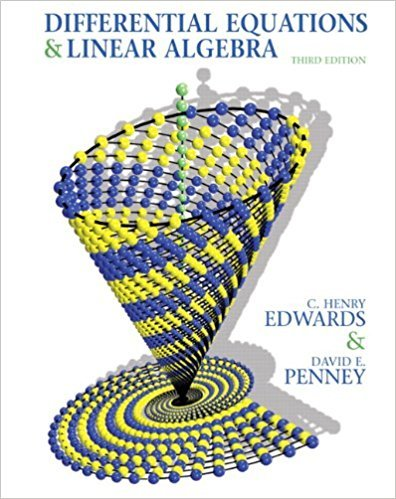×

×

Textbooks / Math / Differential Equations and Linear Algebra 3

# Differential Equations and Linear Algebra 3rd Edition - Solutions by Chapter## Full solutions for Differential Equations and Linear Algebra | 3rd Edition

ISBN: 9780136054252Differential Equations and Linear Algebra | 3rd Edition - Solutions by Chapter

Solutions by Chapter
4 5 0 365 Reviews
##### ISBN: 9780136054252

Differential Equations and Linear Algebra was written by and is associated to the ISBN: 9780136054252. Since problems from 20 chapters in Differential Equations and Linear Algebra have been answered, more than 17538 students have viewed full step-by-step answer. This expansive textbook survival guide covers the following chapters: 20. The full step-by-step solution to problem in Differential Equations and Linear Algebra were answered by , our top Math solution expert on 08/31/17, 10:46AM. This textbook survival guide was created for the textbook: Differential Equations and Linear Algebra, edition: 3.

Key Math Terms and definitions covered in this textbook
• Associative Law (AB)C = A(BC).

Parentheses can be removed to leave ABC.

• Cholesky factorization

A = CTC = (L.J]))(L.J]))T for positive definite A.

• Complete solution x = x p + Xn to Ax = b.

(Particular x p) + (x n in nullspace).

• Diagonalization

A = S-1 AS. A = eigenvalue matrix and S = eigenvector matrix of A. A must have n independent eigenvectors to make S invertible. All Ak = SA k S-I.

• Dimension of vector space

dim(V) = number of vectors in any basis for V.

• Gauss-Jordan method.

Invert A by row operations on [A I] to reach [I A-I].

• Graph G.

Set of n nodes connected pairwise by m edges. A complete graph has all n(n - 1)/2 edges between nodes. A tree has only n - 1 edges and no closed loops.

• Krylov subspace Kj(A, b).

The subspace spanned by b, Ab, ... , Aj-Ib. Numerical methods approximate A -I b by x j with residual b - Ax j in this subspace. A good basis for K j requires only multiplication by A at each step.

• Left inverse A+.

If A has full column rank n, then A+ = (AT A)-I AT has A+ A = In.

• Length II x II.

Square root of x T x (Pythagoras in n dimensions).

• Linear combination cv + d w or L C jV j.

• Minimal polynomial of A.

The lowest degree polynomial with meA) = zero matrix. This is peA) = det(A - AI) if no eigenvalues are repeated; always meA) divides peA).

• Network.

A directed graph that has constants Cl, ... , Cm associated with the edges.

• Norm

IIA II. The ".e 2 norm" of A is the maximum ratio II Ax II/l1x II = O"max· Then II Ax II < IIAllllxll and IIABII < IIAIIIIBII and IIA + BII < IIAII + IIBII. Frobenius norm IIAII} = L La~. The.e 1 and.e oo norms are largest column and row sums of laij I.

• Normal equation AT Ax = ATb.

Gives the least squares solution to Ax = b if A has full rank n (independent columns). The equation says that (columns of A)·(b - Ax) = o.

• Random matrix rand(n) or randn(n).

MATLAB creates a matrix with random entries, uniformly distributed on [0 1] for rand and standard normal distribution for randn.

• Singular Value Decomposition

(SVD) A = U:E VT = (orthogonal) ( diag)( orthogonal) First r columns of U and V are orthonormal bases of C (A) and C (AT), AVi = O'iUi with singular value O'i > O. Last columns are orthonormal bases of nullspaces.

• Skew-symmetric matrix K.

The transpose is -K, since Kij = -Kji. Eigenvalues are pure imaginary, eigenvectors are orthogonal, eKt is an orthogonal matrix.

• Standard basis for Rn.

Columns of n by n identity matrix (written i ,j ,k in R3).

• Vector space V.

Set of vectors such that all combinations cv + d w remain within V. Eight required rules are given in Section 3.1 for scalars c, d and vectors v, w.